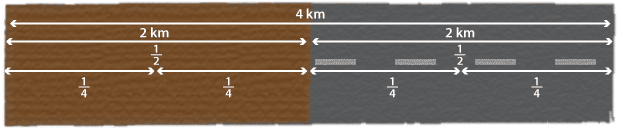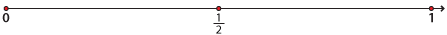## Equivalent fractions

If 2 kilometres of a 4-kilometre road is sealed, we say that $$\dfrac{2}{4}$$ (two quarters) of the road is surfaced. We also know that this means $$\dfrac{1}{2}$$ (half) is sealed. The fractions $$\dfrac{2}{4}$$ and $$\dfrac{1}{2}$$ are called equivalent fractions and we write $$\dfrac{2}{4}=\dfrac{1}{2}$$.### Using a number line

We can say two fractions are equivalent if they are represented by the same marker on a number line.
To show that, for example, $$\dfrac{1}{2}=\dfrac{3}{6}$$ , draw a number line and mark 0 and 1. Form two intervals of equal length from 0 to $$\dfrac{1}{2}$$ and from $$\dfrac{1}{2}$$ to 1. Each interval has a length of $$\dfrac{1}{2}$$.Now divide each of these intervals into three equal subintervals to form six in total. Each of these intervals has a length of $$\dfrac{1}{6}$$.Detailed description

The markers $$\dfrac{1}{2}$$ and $$\dfrac{3}{6}$$ are at the same point on the number line so $$\dfrac{1}{2}$$ and $$\dfrac{3}{6}$$ are equivalent.